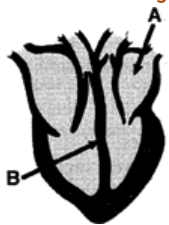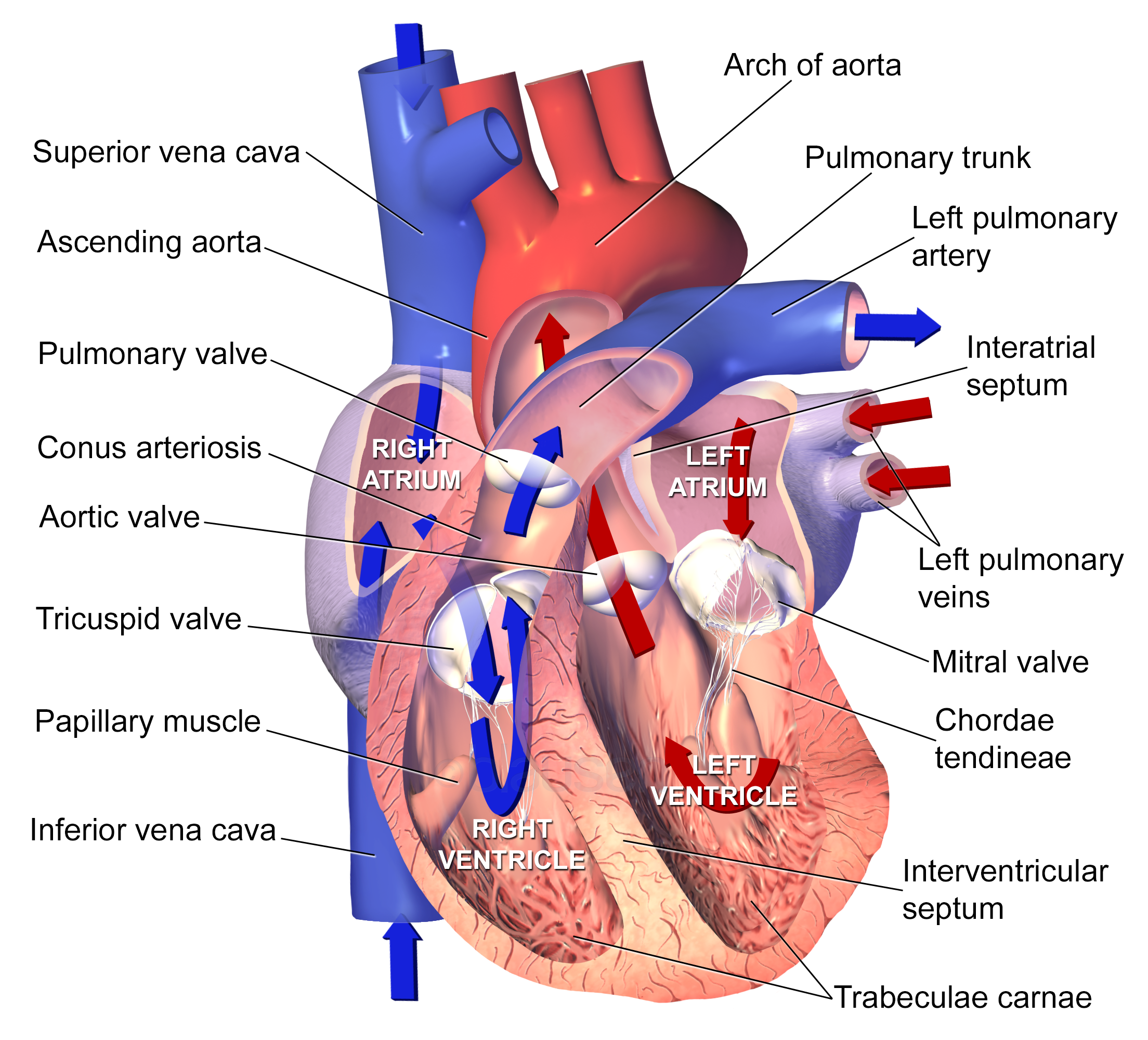(a) A = left atrium
">

# DIRECTIONS: On the basis of the following diagram/ picture answer the questions given below: Study the following diagram carefully and answer the following questions. Name the parts of the heart labeled A and B in the diagram.(a) A = left atrium

Correct Answer(d) A = left atrium, B = septum

Explanation:

Given below is an image of the human heart with its main parts.Updated on: 10-Oct-2022

26 Views